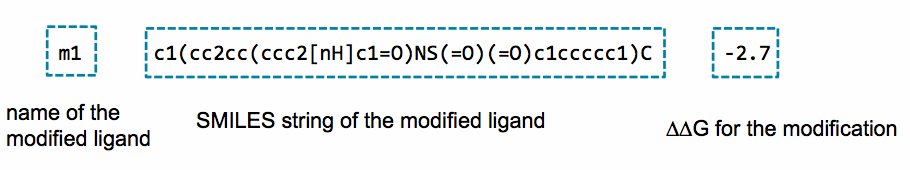# SSFEP: Single Step Free Energy Perturbation¶

## Background¶

Free energy perturbation (FEP) has long been considered the gold standard in calculating relative ligand-binding free energies. However, FEP is often impractical for evaluating large number of changes to a parent ligand due to the large computational cost. Single Step Free Energy Perturbation (SSFEP) is an alternative that can be orders of magnitude faster than conventional FEP when evaluating large number of changes to a parent ligand, while maintaining useful accuracy for small functional group modifications .

The SSFEP method involves post-processing of MD simulation data of a ligand in a given environment in the canonical ensemble to estimate the alchemical free energy change of chemically modifying the ligand. Zwanzig’s FEP formula is used,

(1)$\Delta G_\mathrm{L1 \rightarrow L2}^\mathrm{env} = -k_\mathrm{B}T \ln \left< e^{-\beta \Delta E}\right>_\mathrm{L1}$

where $$k_\mathrm{B}$$ is the Boltzmann constant and $$T$$ is the temperature. The angular brackets indicate an average of the exponential factor over the MD trajectory of ligand $$L1$$ in the given environment, env, which can be either the solvated protein or water. $$\Delta E$$ is the energy difference between the two systems involving L1 and L2, which in practice is computed as the difference in the interaction energies of the two ligands in the corresponding environment:

$\Delta E = E_{L2 - \mathrm{env}} - E_{L1 - \mathrm{env}}$

The environment env in each system is defined as all non-ligand atoms. As the environment is constant between the two ligands, the internal environmental energy cancels exactly during the computation of $$\Delta E$$. In addition, as the difference between L1 and L2 involves a very small number of heavy atom modifications, we expect any differential intra-ligand energy terms to also cancel exactly between the solution and protein environments. Therefore, once $$\Delta G_{L1\rightarrow L2}^\mathrm{protein}$$ and $$\Delta G_{L1\rightarrow L2}^\mathrm{water}$$ are computed according to Eq. (1), the relative binding free energy is given by

$\Delta \Delta G_{L1\rightarrow L2}^\mathrm{bind} = G_{L1\rightarrow L2}^\mathrm{protein} - G_{L1\rightarrow L2}^\mathrm{water}$

The SSFEP approach allows the data from simulation of a single protein-ligand complex to be rapidly post-processed to evaluate tens to hundreds of potential modifications involving multiple sites on the parent ligand. Given this, the best results are achieved when SSFEP is used to evaluate small modifications to the parent ligand.

In a recent study , the ability of standard FEP and SSFEP to reproduce the experimental relative binding affinities of known ligands for two proteins, ACK1 and p38 MAP kinase, was tested. SSFEP was able to produce comparable results to full FEP while requiring a small fraction of the computational resources.

## Running SSFEP from the SilcsBio GUI¶

Please see SSFEP simulation from the GUI in the Graphical User Interface Quickstart for instructions on running SSFEP from the SilcsBio GUI.

## Running SSFEP from the command line interface¶

The following usage details are provided for completeness. We strongly recommend using the SilcsBio GUI to set up, run, and analyze SSFEP calculations.

To perform the SSFEP precomputation simulations, protein coordinates in PDB file format and parent ligand coordinates in Mol2 file format are required. The protein should have termini properly capped, missing loops built or the ends of the missing loops capped, standard atom and residue names, and sequential atom and residue numbering. Using these two files, run the following:

${SILCSBIODIR}/ssfep/1_setup_ssfep prot=<Protein PDB> lig=<Ligand Mol2/SDF>  Warning The setup program internally use the GROMACS utility pdb2gmx, which may have problems processing the protein PDB file. The most common pdb2gmx issue involves mismatches between the expected residue name/atom names in the input PDB and those defined in the CHARMM force-field. To fix this problem: Run the pdb2gmx command manually from within the 1_setup directory for a detailed error message. Please contact support@silcsbio.com for additional assistance. Following completion of the setup, run 10 MD jobs: ${SILCSBIODIR}/ssfep/2_run_md_ssfep prot=<Protein PDB> lig=<Ligand Mol2/SDF>


This command will submit 10 jobs to the pre-defined queue: 5 for the ligand in water and 5 for the ligand complexed with protein.

Once the precomputation simulations are completed, the 2_run_md/1_lig/[1-5] and 2_run_md/2_prot_lig/[1-5] directories will contain *.1-10.whole.trr trajectory files. If these files are not generated, then your simulations are either still running or have stopped due to a problem. Look into the log files within these directories for an explanation of the failure.

## Ligand modifications¶

Follow the instructions in Chemical group transformations to create modifications to your parent ligand.

## Evaluating binding affinity changes¶

Once modifications.txt has been prepared and the MD simulations involving the parent ligand are completed, run the following script to set up a $$\Delta \Delta G$$ calculation.

${SILCSBIODIR}/ssfep/3a_setup_modifications prot=<Protein PDB> lig=<Ligand Mol2/SDF File> mod=modifications.txt  This will submit 10 jobs to evaluate all snapshots from the completed MD simulations of the parent ligand in order to calculate the change in free energy for every modification specified in your modifications.txt. Structures of these modifications in mol2 format are output as 3_analysis_<modified ligand name entry in modifications.txt>/mod_files/*.mol2. After these jobs complete, you may obtain $$\Delta \Delta G$$ for your full list of modifications using: ${SILCSBIODIR}/ssfep/3b_calc_ddG_ssfep mod=modifications.txt


Example output follows: